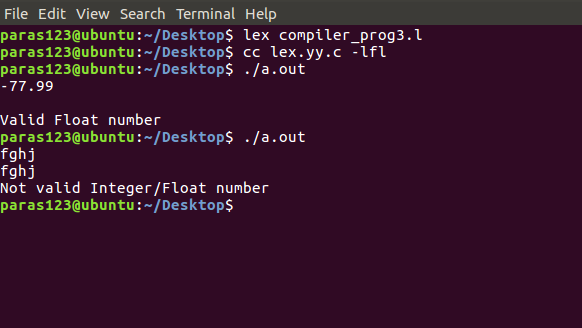# Lex Program to accept a valid integer and float value

Lex is a computer program that generates lexical analyzers.
Lex reads an input stream specifying the lexical analyzer and outputs source code implementing the lexer in the C programming language.

The commands for executing the lex program are:

```lex abc.l (abc is the file name)
cc lex.yy.c -efl
./a.out
```

Let’s see to accept a valid integer and float value
using lex program.

Examples:

```Input :
-77.99
Output :
Valid Float Value

Input :
fghj
Output :
Not a valid Integer/ Float number
```

Below is the implementation:

 `/*lex code to accept a valid integer  ` `  ``and float value using lex program.*/` `   `  `%{ ` `int` `valid_int=0, valid_float=0; ` `%} ` ` `  `%% ` `^[-+]?[0-9]* valid_int++; ` `^[-+]?[0-9]*[.][0-9]+\$ valid_float++; ` `.; ` `%% ` ` `  `int` `main() ` `{ ` `  ``yylex(); ` `  ``if``(valid_int!=0) ``printf``(``"Valid Integer number\n"``); ` `  ``else` `if``(valid_float!=0) ``printf``(``"Valid Float number\n"``); ` `  ``else` `printf``(``"Not valid Integer/Float number\n"``); ` `  ``return` `0; ` `} `

Output:Whether you're preparing for your first job interview or aiming to upskill in this ever-evolving tech landscape, GeeksforGeeks Courses are your key to success. We provide top-quality content at affordable prices, all geared towards accelerating your growth in a time-bound manner. Join the millions we've already empowered, and we're here to do the same for you. Don't miss out - check it out now!

Previous
Next